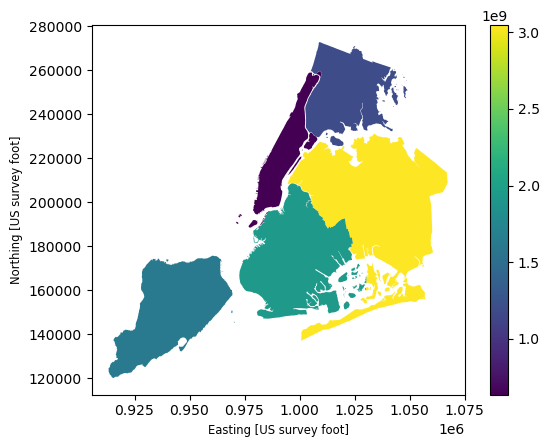# Introduction to GeoPandas#

This quick tutorial introduces the key concepts and basic features of GeoPandas to help you get started with your projects.

## Concepts#

GeoPandas, as the name suggests, extends the popular data science library pandas by adding support for geospatial data. If you are not familiar with pandas, we recommend taking a quick look at its Getting started documentation before proceeding.

The core data structure in GeoPandas is the geopandas.GeoDataFrame, a subclass of pandas.DataFrame, that can store geometry columns and perform spatial operations. The geopandas.GeoSeries, a subclass of pandas.Series, handles the geometries. Therefore, your GeoDataFrame is a combination of pandas.Series, with traditional data (numerical, boolean, text etc.), and geopandas.GeoSeries, with geometries (points, polygons etc.). You can have as many columns with geometries as you wish; there’s no limit typical for desktop GIS software.Each GeoSeries can contain any geometry type (you can even mix them within a single array) and has a GeoSeries.crs attribute, which stores information about the projection (CRS stands for Coordinate Reference System). Therefore, each GeoSeries in a GeoDataFrame can be in a different projection, allowing you to have, for example, multiple versions (different projections) of the same geometry.

Only one GeoSeries in a GeoDataFrame is considered the active geometry, which means that all geometric operations applied to a GeoDataFrame operate on this active column.

User guide

See more on data structures in the user guide.

Let’s see how some of these concepts work in practice.

First, we need to read some data.

Assuming you have a file containing both data and geometry (e.g. GeoPackage, GeoJSON, Shapefile), you can read it using geopandas.read_file(), which automatically detects the filetype and creates a GeoDataFrame. This tutorial uses the "nybb" dataset, a map of New York boroughs, which is available through the geodatasets package. Therefore, we use geodatasets.get_path() to download the dataset and retrieve the path to the local copy.

:

import geopandas
from geodatasets import get_path

path_to_data = get_path("nybb")

gdf

ERROR 1: PROJ: proj_create_from_database: Open of /home/docs/checkouts/readthedocs.org/user_builds/geopandas/conda/latest/share/proj failed

:

BoroCode BoroName Shape_Leng Shape_Area geometry
0 5 Staten Island 330470.010332 1.623820e+09 MULTIPOLYGON (((970217.022 145643.332, 970227....
1 4 Queens 896344.047763 3.045213e+09 MULTIPOLYGON (((1029606.077 156073.814, 102957...
2 3 Brooklyn 741080.523166 1.937479e+09 MULTIPOLYGON (((1021176.479 151374.797, 102100...
3 1 Manhattan 359299.096471 6.364715e+08 MULTIPOLYGON (((981219.056 188655.316, 980940....
4 2 Bronx 464392.991824 1.186925e+09 MULTIPOLYGON (((1012821.806 229228.265, 101278...

### Writing files#

To write a GeoDataFrame back to file use GeoDataFrame.to_file(). The default file format is Shapefile, but you can specify your own with the driver keyword.

:

gdf.to_file("my_file.geojson", driver="GeoJSON")


User guide

See more on reading and writing files in the user guide.

## Simple accessors and methods#

Now we have our GeoDataFrame and can start working with its geometry.

Since there was only one geometry column in the New York Boroughs dataset, this column automatically becomes the active geometry and spatial methods used on the GeoDataFrame will be applied to the "geometry" column.

### Measuring area#

To measure the area of each polygon (or MultiPolygon in this specific case), access the GeoDataFrame.area attribute, which returns a pandas.Series. Note that GeoDataFrame.area is just GeoSeries.area applied to the active geometry column.

But first, to make the results easier to read, set the names of the boroughs as the index:

:

gdf = gdf.set_index("BoroName")

:

gdf["area"] = gdf.area
gdf["area"]

:

BoroName
Staten Island    1.623822e+09
Queens           3.045214e+09
Brooklyn         1.937478e+09
Manhattan        6.364712e+08
Bronx            1.186926e+09
Name: area, dtype: float64


### Getting polygon boundary and centroid#

To get the boundary of each polygon (LineString), access the GeoDataFrame.boundary:

:

gdf["boundary"] = gdf.boundary
gdf["boundary"]

:

BoroName
Staten Island    MULTILINESTRING ((970217.022 145643.332, 97022...
Queens           MULTILINESTRING ((1029606.077 156073.814, 1029...
Brooklyn         MULTILINESTRING ((1021176.479 151374.797, 1021...
Manhattan        MULTILINESTRING ((981219.056 188655.316, 98094...
Bronx            MULTILINESTRING ((1012821.806 229228.265, 1012...
Name: boundary, dtype: geometry


Since we have saved boundary as a new column, we now have two geometry columns in the same GeoDataFrame.

We can also create new geometries, which could be, for example, a buffered version of the original one (i.e., GeoDataFrame.buffer(10)) or its centroid:

:

gdf["centroid"] = gdf.centroid
gdf["centroid"]

:

BoroName
Staten Island     POINT (941639.450 150931.991)
Queens           POINT (1034578.078 197116.604)
Brooklyn          POINT (998769.115 174169.761)
Manhattan         POINT (993336.965 222451.437)
Bronx            POINT (1021174.790 249937.980)
Name: centroid, dtype: geometry


### Measuring distance#

We can also measure how far each centroid is from the first centroid location.

:

first_point = gdf["centroid"].iloc
gdf["distance"] = gdf["centroid"].distance(first_point)
gdf["distance"]

:

BoroName
Staten Island         0.000000
Queens           103781.535276
Brooklyn          61674.893421
Manhattan         88247.742789
Bronx            126996.283623
Name: distance, dtype: float64


Note that geopandas.GeoDataFrame is a subclass of pandas.DataFrame, so we have all the pandas functionality available to use on the geospatial dataset — we can even perform data manipulations with the attributes and geometry information together.

For example, to calculate the average of the distances measured above, access the ‘distance’ column and call the mean() method on it:

:

gdf["distance"].mean()

:

76140.09102166798


## Making maps#

GeoPandas can also plot maps, so we can check how the geometries appear in space. To plot the active geometry, call GeoDataFrame.plot(). To color code by another column, pass in that column as the first argument. In the example below, we plot the active geometry column and color code by the "area" column. We also want to show a legend (legend=True).

:

gdf.plot("area", legend=True)

:

<Axes: >You can also explore your data interactively using GeoDataFrame.explore(), which behaves in the same way plot() does but returns an interactive map instead.

:

gdf.explore("area", legend=False)

:

Make this Notebook Trusted to load map: File -> Trust Notebook